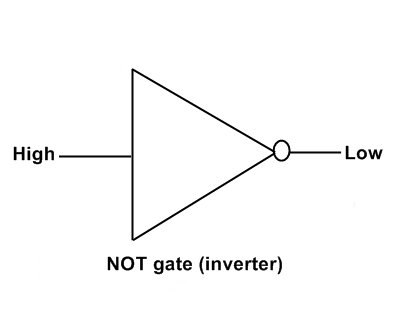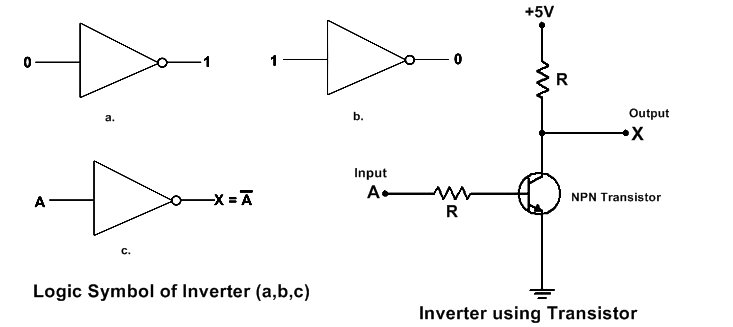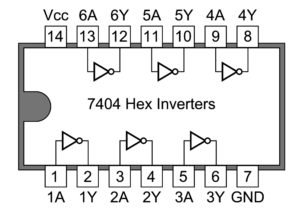### What is NOT gate (Inverter)

NOT gate is Device which gives output always the inverse of INPUT. NOT gate output is always the complement of its INPUT. NOT gate also called an INVERTER and which has only one input and only one output. The main function of inverter (NOT gate) is the invert the input signal at ouput. Output signal is inverted of input signal. Low input gives a high output and vice versa.

• In the NOT gate if input is 1 then the output is 0 and if the input is 0 then its output is 1. Here 1 means the Positive supply or High state. 0 means 0v supply or LOW state.
• We assume that in any NOT gate circuit we connect the input +5v , this +5v input is HIGH and Logically 1. Then the output of it is 0v means Logically 0 .
• At input If there is 0v supply . It means input is 0 (LOW) then the output of NOT gate is +ve (HIGH) . Logically 1, and here we use 5v then the output is 5v.
• In other word When input is OFF then output is get ON and if Input is ON then Output remain OFF.The output of NOT gate is represented by A- (A bar) . If input is A and output is X then the expression for the output is X=A- .
Not gate may be made using connect of one transistor and two resistor as shown in fig. This method of Realization of gate is called RTL (Resistor transistor Logic).
When Input is 0v then the transistor is OFF and output which is connected with the collector get current through the resistor R2. When Switch ON and there is a supply Given on Base of transistor then the transistor is Turn ON and current passed between emitter and collector and All voltage is dropped on R2 which is come from VCC and therefore the output is 0v, means output get turn Off.

#### NOT gate Inverter Logic circuit using transistor

In one chip there are NOT Gate include in single IC . The IC 7404 is made for operation of NOT gate (INVERTER) . 7404 ic has 6 NOT gate. The IC 74LS04 is Hex inverter IC. These IC contain six independent inverters.